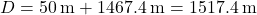## A 3-kg projectile is launched at an angle of 45o above the horizontal. The projectile explodes at the peak of its flight into two pieces. A

Question

A 3-kg projectile is launched at an angle of 45o above the horizontal. The projectile explodes at the peak of its flight into two pieces. A 2-kg piece falls directly down and lands exactly 50 m from the launch point. Determine the horizontal distance from the launch point where the 1-kg piece lands.

in progress 0
8 months 2021-07-17T09:27:36+00:00 1 Answers 1 views 0

1. 1517.4 m

Step-by-step explanation:

Since the projectile broke up at the peak of its flight, it already traveled half its initial range so we can find its initial launch velocity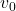from the equation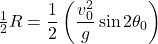where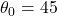and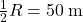so we will get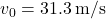. Next, we can use the equation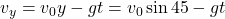and since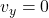at its peak, we get t = 22.1 s. Let’s set this aside for a moment and we’ll use it later.

At the top of its peak, we can use the conservation law of linear momentum. Let M be the mass if of the original projectile,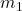be the mass of the larger fragment (2 kg) and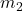be the mass of the smaller fragment (1 kg). We can write the conservation law as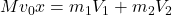where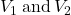are the velocities of the fragments immediately after the break up. But we also know that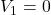so the velocity ofcan be calculated from the conservation law as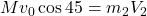or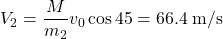Now we can calculate the horizontal distance the smaller fragment traveled after the break up. Recall that the amount of time for it to go up is also the amount of time to get down so the horizontal distance x is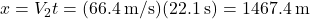Therefore, the total distance traveled from the launch point is